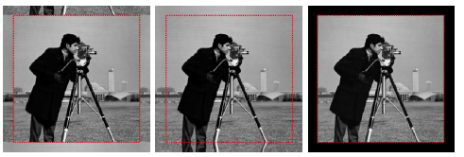# Deconvolving Images with Unknown Boundaries Using the Alternating Direction Method of Multipliers Synopsis

Download 112.54 Kb.
 Date 23.04.2018 Size 112.54 Kb.
 Deconvolving Images with Unknown Boundaries Using the Alternating Direction Method of Multipliers Synopsis The alternating direction method of multipliers (ADMM) has recently sparked interest as a flexible and efficient optimization tool for imaging inverse problems, namely deconvolution and reconstruction under non-smooth convex regularization. ADMM achieves state-of-the-art speed by adopting a divide and conquer strategy, wherein a hard problem is split into simpler, efficiently solvable sub-problems (e.g., using fast Fourier or wavelet transforms, or simple proximity operators). In deconvolution, one of these sub-problems involves a matrix inversion (i.e., solving a linear system), which can be done efficiently (in the discrete Fourier domain) if the observation operator is circulant, i.e., under periodic boundary conditions. This paper extends ADMM-based image deconvolution to the more realistic scenario of unknown boundary, where the observation operator is modeled as the composition of a convolution (with arbitrary boundary conditions) with a spatial mask that keeps only pixels that do not depend on the unknown boundary. The proposed approach also handles, at no extra cost, problems that combine the recovery of missing pixels (i.e., inpainting) with deconvolution. We show that the resulting algorithms inherit the convergence guarantees of ADMM and illustrate its performance on non periodic deblurring (with and without inpainting of interior pixels) under total-variation and frame-based regularization. Introduction The alternating direction method of multipliers (ADMM),originally proposed in the 1970’semerged recently as flexible and efficient tool for several imaging inverse problems, such as denoising deblurring inpainting ,reconstruction, motion segmentation, to mention only a few classical problems (for a comprehensive review, see ). ADMM-based approaches make use of variable splitting, which allows a straightforward treatment of various priors/regularizes , such as those based on frames or on total-variation (TV) , as well as the seamless inclusion of several types of constraints (e.g., positivity) , .ADMM is closely related to other techniques, namely the socalledBregman and split Bregman methods]and Douglas-Rachford splitting .Several ADMM-based algorithms for imaging inverse problemsrequire, at each iteration, solving a linear system (equivalently,inverting a matrix) . This fact is simultaneously a blessing and a curse. On the onehand, the matrix to be inverted is related to the Hessian of the objective function, thus carrying second order information;arguably, this fact justifies the excellent speed of these methods,which have been shown (see, e.g., ) to be considerablyfaster than the classical iterative shrinkage-thresholding (IST)algorithms and even than their accelerated versions. On the other hand, this inversion (due to itstypically huge size) limits its applicability to problems where it can be efficiently computed (by exploiting some particular structure). For ADMM-based image deconvolution algorithms , this inversion can be efficiently carried out using the fast Fourier transform (FFT), if the convolution is cyclic/periodic (or assumed to be so), thus diagonal in the discrete Fourier domain. However, as explained next, periodicity is an unnatural assumption, inadequate for most real imaging problems. In deconvolution, the pixels located near the boundary of the observed image depend on pixels (of the unknown image) located outside of its domain. The typical way to formalize this issue is to adopt a so-called boundary condition (BC). • The periodic BC (the use of which, in image deconvolution, dates back to the 1970s ) assumes a periodic convolution; its matrix representation is circulant1, diagonalized by the DFT1, which can be implemented via the FFT. This computational convenience makes it, arguably, the most commonly adopted BC. • The zero BC assumes that all the external pixels have zero value, thus the matrix representing the convolution is block-Toeplitz, with Toeplitz blocks. By analogy with the BC for ordinary or partial differential equations that assumes fixed values at the domain boundary, this is commonly referred to Dirichlet BC. • In the reflexive and anti-reflexive BCs, the pixels outside the image domain are a reflection of those near the boundary, using even or odd symmetry, respectively. In the reflexive BC, the discrete derivative at the boundary is zero; thus, by analogy with the BC for ordinary or partial differential equations that assumes fixed values of the derivative at the boundary, the reflexive BC is often referred to as Neumann BC.Illustration of the (unnatural) assumptions underlying the periodic, reflexive, and zero boundary conditions. References  M. V. Alonso, J. M. Bivouacs-Dias, and M. A. T. Figueiredo, “An augmented Lagrangian approach to linear inverse problems with compound regularization,” in IEEE Intern. Conf. on Image Processing – ICIP, 2010.  “Fast image recovery using variable splitting and constrained optimization,” IEEE Trans. on Image Processing, vol. 19, pp. 2345–2356, 2010.  “An augmented Lagrangian approach to the constrained optimization formulation of imaging inverse problems,” IEEE Transactions on Image Processing, vol. 20, pp. 681–695, 2011.  ——, “Non-cyclic deconvolution using an augmented Lagrangian method,” in IEEE International Conference on Computer as a Tool (EUROCON), 2011, pp. 1–4.  M. Almeida and M. A. T. Figueiredo, “Blind deblurring of images with unknown boundaries using the alternating direction method of multipliers,” 2013, submitted.Download 112.54 Kb.Share with your friends:

The database is protected by copyright ©ininet.org 2020
send message

Main page